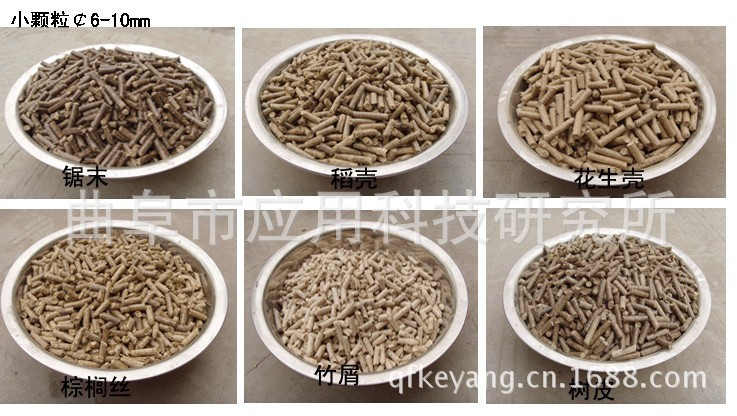﻿ 山东机械， 颗粒机械 科阳机械, 木质颗粒机械_涂料网_采购中心_工业制造网客服热线：15105419926

# 山东机械， 颗粒机械 科阳机械, 木质颗粒机械

• 需求数量：20

### 采购样品图

•••• ### 采购详情

r

r              “科阳”牌燃料造粒机**的机器 r

r

r
r

r

r     r

r

r     木粉制粒机又名生物质燃料颗粒加工设备。 r

r

r     在能源日益紧缺、环境不断恶化的**，生物质燃料颗粒成为煤、石油、天然气的**替代品，是一种高效、洁净的可再生能源。 r

r

r     生物质颗粒加工设备可以将各锯木屑、（玉米、麦草、稻草、棉花等）秸秆、花生壳、葵花籽壳、棕榈壳、稻壳、甘蔗渣、咖啡渣、中药渣、糠醛渣、蓖麻壳、树枝树皮、及经分拣的工业垃圾等原料挤压成型直径6-10mm的柱状可燃料颗粒。生物质燃料颗粒加工机设备的生产与推广具有良好的经济效益和社会效益。 r

r

r     制粒优势：     r

r

r     1.减少粉尘污染环境，合理利用废弃资源； r

r

r     2.方便贮存，节省存放空间（约7立方原料产生1立方颗粒），体积压缩，便于运输； r

r

r     3.原料被挤压成颗粒燃料时热燃料值提高，灰分降低； r

r

r     6.干法制，原料无须特殊处理，成型冷却后便是成品。 r

r

r     设备特点： r

r

r     1．变频调速喂料，入料速度可调，利于设备平衡运转； r

r

r     2．垂直进料，物料直接进入压制室，进料无阻塞； r

r

r     3．模具静止，压辊旋转，物料离心，均布模具四周； r

r

r     4．更换模具，便可压制不同规格的产品； r

r

r     5．环形模具，立向结构，利于制粒室散热降温； r

r

r     6．压轮与模具装配到位，保证颗粒的成型率。 r

r

r     结构原理：设备压制室包括沿传动轴轴向对称的可转动碾压辊，碾压辊的外周向上有环模，环模的环模径向上分布着内大外小的锥形孔，环模的外周有周向转动的切粒刀，切粒刀的外周有环模罩，环模罩的上部接有进料口，下面接有出料口。工作时碾压辊沿环模内周旋转碾压来自于进料口的原料，使原料进入环模上分布的沿径向内大外小的锥形孔，原料在锥形孔中不断受到挤压向外挤出成密实柱体，经切粒刀切断成柱型颗粒。 r

r

r     技术参数： r

r r r r r r r r r r r r r r r r r r r r r r r r
 r r 设备型号 r r r r 93KWH-350(立) r r r r 93KWH-420（立） r r r r 匹配动力（kw） r r r r 55+0.55 r r r r 75+0.55 r r r r 产量（kg/h） r r r r 500-800 r r r r 1000-1500 r r r r 设备配置 r r r r 螺旋输送机、贮料仓、变频振动喂料器、制粒主机 r r
r r

rr

r

r
r

r

## 采购商资料

登录后方可见采购商详细资料